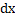# A uniform chain of lengthand massis lying on a smooth table and one–third of its length is hanging vertically down over the edge of the table. Ifis the acceleration due to gravity, the work required to pull the hanging part on to the table is a)b)c)d)## Question ID - 100656 :- A uniform chain of lengthand massis lying on a smooth table and one–third of its length is hanging vertically down over the edge of the table. Ifis the acceleration due to gravity, the work required to pull the hanging part on to the table is a)b)c)d)3537

(d)

Method I:

The hanging part of the chain which is to be pulled can be considered as a point mass situated at the centre of the hanging part. The equivalent diagram is drawn The work done in bringing the mass up will be equal to the change in potential energy of the massChange in potential energyMethod II:

The mass per unit of the chainLet us consider a finitesimally small length of the chainat a distancefrom the bottom. To moveto the top, a force equal to the weight of chainwill have to be applied upwardsWeight of chain of lengthSmall amount of work done in movingto the topThe total amount of work done in moving the one-third length of the hanging chain on the table will beNext Question :

In question the numbers/letters are arranged in a sequence based on certain principle. Select the answer from the four alternatives given under each sequence for the term marked by ‘?’.

 23, 35, 57, 711, ? (a) 213 (b) 1311 (c) 1113 (d) 1317